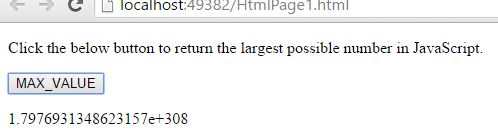# MAX_VALUE Property in JavaScript

##### How to return the largest number possible in JavaScript?

By using `MAX_VALUE` property we can return the largest number which is possible in JavaScript.

```<p>Click the below button to return the largest possible number in JavaScript.</p>
<input type="button" value="MAX_VALUE" onclick="Function()" />
<p id="myId"></p>

<script>
function Function() {
document.getElementById("myId").innerHTML = Number.MAX_VALUE;
}
</script>
```

In the above code snippet we have given `Id` as "`myId`"to the second `<p>` element in the HTML code, we need to return the largest number possible in JavaScript. For that we are using the code `Number.MAX_VALUE`. There is a Function() in the`<script>`block which is connected to the Onclick of the HTML button. The `document.getElementById(`"myId"`) ` returns the element that has Id "id=myID" in the HTML page. Onclick of the button "MAX_VALUE" fires the Function() in the `<script>` block at the same time `Number.MAX_VALUE `returns the largest number possible in JavaScript and gives the output.

OUTPUTNOTE: In the above output, the value of e=10, there are many numbers which are larger than MAX_VALUE, but those are all represented as infinity in JavaScript.

Views: 3478 | Post Order: 82